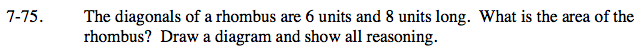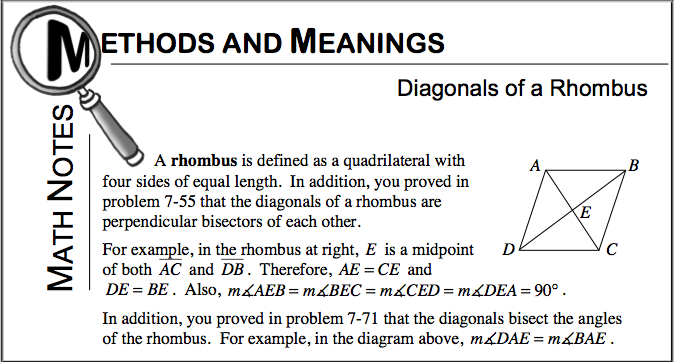### Home > GC > Chapter 7 > Lesson 7.2.4 > Problem7-75

7-75.

The diagonals of a rhombus are 6 units and 8 units long. What is the area of the rhombus? Draw a diagram and show all reasoning. Homework Help ✎Refer to the Math Notes box below from Lesson 7.2.4.To ''bisect'' means to divide into two equal parts. Perpendicular objects form 90° angles.